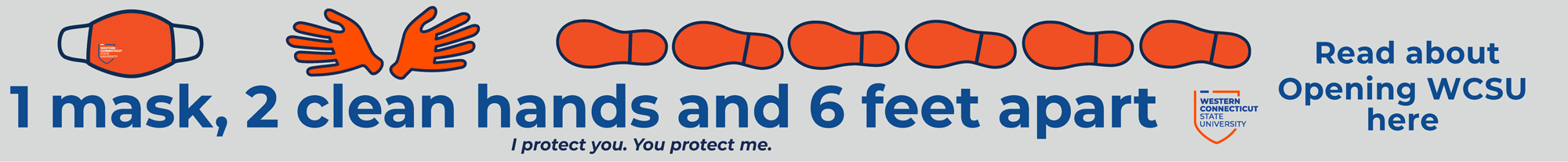# Math Department

Bachelor of Science In Applied and Computational Mathematics

One Degree – Three Options

The B.S. in Applied and Computational Mathematics is one degree that consists of the following three options that a student may choose from:

1. Mathematics of Data Science and Machine Learning
2. Actuarial Science
3. Applied Differential Equations and Scientific Computing

Requirements:

In addition to the courses listed below, students must complete all university general education requirements and sufficient additional free electives to total a minimum of 120 semester hours, including foreign language and physical education.

All Option Areas Must Take

• MAT 141 – Foundational Discrete Math
• MAT 150 – Math Seminar I
• MAT 151 – Math Seminar II
• MAT 181 – Calculus I
• MAT 182 – Calculus II
• MAT 207 – Proofs
• MAT 222 – Introductory Statistics
• MAT 272 – Linear Algebra
• MAT 281 – Calculus III
• MAT 282 – Ordinary Differential Equations
• MAT 322 – Probability
• MAT 332 – Applied Linear Algebra and Math of Machine Learning
• MAT 380 – Math Modeling with Symbolic and Scientific Computations
• MAT 383 – Introduction to Mathematical Analysis
• MAT 453 – Senior Seminar (CE,W3) (OR SIS with Project) (OR Senior Thesis) (OR Internship)

Further Requirements (Option Specific)

Mathematics of Data Science and Machine Learning Option:

Further Required MAT Courses

• MAT 422 – Statistics for Data/Actuarial Science and Machine Learning
• MAT 470 – Applications of Machine Learning and Wavelets

One of:

• MAT 468 – Partial Differential Equations
• MAT 469 – Numerical Methods for Ordinary and Partial Differential Equations (OPDEs)

Cognates

• PHI 227 – Ethics in Computing
• CS 140 – Introduction to Programming (Python)

Application Area Courses

• CS 172 – Intermediate Java Programming
• CS 205 – Data Modeling and Database Design
• CS 250 – Introduction to Data Structures, Algorithms and Complexity
• CS 303 – Introduction to Data Science with Python

Applied Differential Equations and Scientific Computing Option:

Currently, students interested in this option will need to pick one of the following sequences to pursue: a chemistry sequence (CHE) or meteorology sequence (MTR).  For students interested in other application areas,  with departmental and university approval, we hope to add a sequence in biology as well as other areas of interest. Consult with Drs Christofi, Shoushani, or Wang.

Further Required MAT Courses

Regardless of the sequence pursued, students interested in this option must take:

• MAT 468 – Partial Differential Equations
• MAT 469 – Numerical Methods for Ordinary and Partial Differential Equations (OPDEs)
• MAT 470 – Applications of Machine Learning and Wavelets

Cognates

Cognates (Sequence CHE)
CS 140 – Introduction to Programming (Python)

Cognates (Sequence MTR)

• CS 140 – Introduction to Programming (Python)
• PHY 110 – General Physics I (Calculus)

Application Area Courses

Sequence CHE
Lecture only:

• CHE 110 – General Chemistry I
• CHE 111 – General Chemistry II
• CHE 300 – Physical Chemistry I
• CHE 301 – Physical Chemistry II

Sequence MTR

• PHY 111 – General Physics II (Calculus),
• MTR 310 – Atmospheric Thermodynamics,
• MTR 311 – Atmospheric Dynamics,
• MTR 340 – Mesoscale Meteorology and Numerical Forecasting

Actuarial Science Option:

Further Required MAT Courses

• MAT 329 – Actuarial Mathematics
• MAT 422 – Statistics for Data/Actuarial Science and Machine Learning

One of:

• MAT 468 – Partial Differential Equations
• MAT 469 – Numerical Methods for Ordinary and Partial Differential Equations (OPDEs)
• MAT 470 – Applications of Machine Learning and Wavelets

Cognates

• CS 143 – Visual BASIC

Application Area Courses

• ACC 201 – Financial Accounting
• ECO 211 – Principles of Macroeconomics
• ECO 213 – Principles of Microeconomics
• FIN 310 – Principles of Finance

Learning Outcomes:

The BS in Applied and Computational Math program will graduate students who will:

1.  Demonstrate possession of a resilient mathematical foundation that allows them to reason rigorously in mathematical arguments and that is adaptable to current and future trends. This foundation encompasses the core areas of:

a. Real and numerical analysis
b. Differential equations
c. Linear algebra
d. Probability and statistics

2. Connect different areas of mathematics with other disciplines and demonstrate proficiency in at least one of the following modern applications:

a. Data Science and Machine Learning
b. Scientific Computing (coupled with a specific discipline/application area)
c. Actuarial Science.

3. Demonstrate an ability to synthesize and apply major theoretical and/or computational techniques and concepts to analyze, construct, and solve realistic models of practical importance and:

a. Recognize the limitations of the theoretical concepts in building solutions to real-world problems

b. Adapt theoretical ideas to develop efficient numerical solutions to real-world problems

c. Adapt theoretical ideas to develop efficient algorithms that can be applied to real-world problems.

4. Use relevant software and technology (such as MATLAB, Mathematica, Python, and LaTeX) and/or write computer programs to construct, visualize, analyze, and interpret solutions to applied mathematical problems.

5. Be able to, working independently or collaboratively, apply concepts learned either from relevant coursework, possible internships, or research projects with faculty, to write mathematical reports that effectively communicate findings to others (for instance, other classmates and/or attendees at local/international conferences or research symposia such as Western Research Day), and that can serve as the basis for possible publications.

Program Sheets

Prerequisite Flowcharts

Four Year Plan of Study:

Four year plans for each of the options can be found at the links below